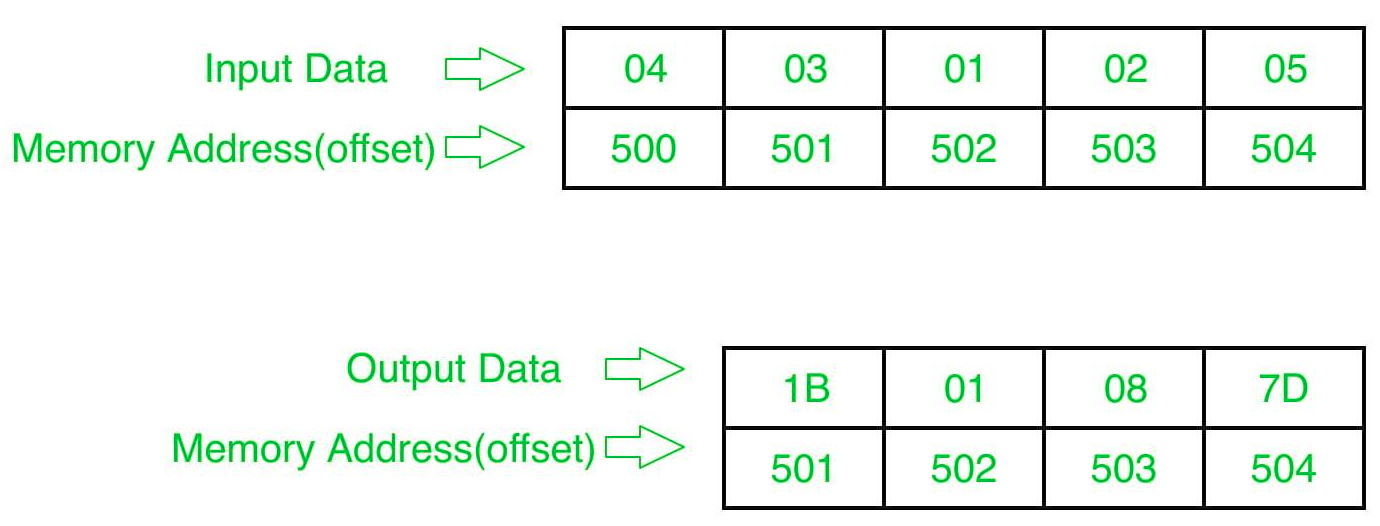# 8086 program to determine cubes of numbers in an array of n numbers

• Last Updated : 19 Jun, 2018

Problem – Write a program in 8086 microprocessor to find out the cubes of 8-bit n numbers, where size “n” is stored at offset 500 and the numbers are stored from offset 501 and store the result numbers into offset 501.(assuming cubes comes out to be in limit of 8 bit only).

Example –Algorithm –

1. Store 500 to SI and Load data from offset 500 to register CL and set register CH to 00 (for count).
2. Increase the value of SI by 1.
3. Load number(value) from offset SI to register AL.
4. Move the value of register AL to BL.
5. Multiply the value in register AL by itself.
6. Multiply the value in register AL by BL.
7. Store the result (value of register AL ) to memory offset SI.
8. Increase the value of SI by 1.
9. Loop above 6 till register CX gets 0.

Program –

400 MOV SI, 500 SI<-500
403 MOV CL, [SI] CL<-[SI]
405 MOV CH, 00 CH<-00
407 INC SI SI<-SI+1
408 MOV AL, [SI] AL<-[SI]
40A MOV BL, AL BL<-AL
40C MUL AL AX=AL*AL
40E MUL BL AX=AL*BL
410 MOV [SI], AL AL->[SI]
412 INC SI SI<-SI+1
415 HLT end

Explanation –

1. MOV SI, 500: set the value of SI to 500
2. MOV CL, [SI]: load data from offset SI to register CL
3. MOV CH, 00: set value of register CH to 00
4. INC SI: increase value of SI by 1.
5. MOV AL, [SI]: load value from offset SI to register AL
6. MOV BL, AL: move value of register AL to BL.
7. MUL AL: multiply value of register AL by AL.
8. MUL BL: multiply value of register AL by BL.
9. MOV [SI], AL: store value of register AL at offset SI.
10. INC SI: increase value of SI by 1.